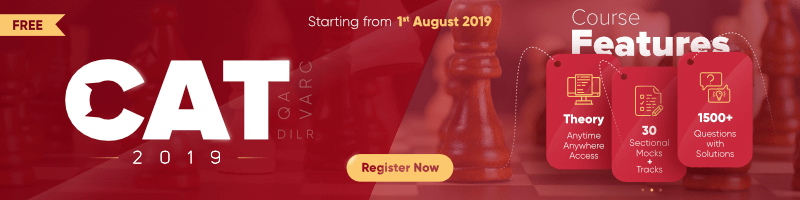# Permutation and Combination | Set-2

Question 1: How many number greater than ten thousand can be formed from the digits 0, 1, 2, 3, 4 without repetation ?
Solution : In order to form a number greater than 10000 we should have only 5 digits. Since, we have 5 digits we cannot take 0 in starting position.
_ _ _ _
For first digit, we have 4 choices.
For second digit, again we have 4 choices because we can include 0 from here onwards.
For third digit, we have 3 choices.
and for fourth digit only left 2 choices.
Total numbers = 4x4x3x2= 96
Hence, only 96 numbers possible.

Question 2: In how many ways can 4 boys and 4 girls be seated around a circular table so that no two boys are in adjacent positions?
Solution : If we first put 4 boys around the table, we can do this in 3! ways.
Once the 4 boys are placed, we have to place 4 girls around the same table.
Now, we can see 4 vacant places are there between all 4 boys so we can do in 4! ways.
Total number of sitting arrangement = 3! x 4!

Question 3: Out of the 11 points in a plane, 4 are collinear. How many straight line can be formed ?
Solution : If all points were non-collinear then possible lines would have been 11C2.
But, 4 points are collinear lie on the same line. So, they are all counted as a single line.
Total number of straight line = 11C24C2 + 1
= 11×10/2 – 4×3/2 + 1
= 55 – 6 + 1
= 50

Question 4: Twenty people attend a party and shake hands with one another.In how many ways hand shake is possible?
Solution : All people shake hands with one another except himself.
1st person has 19 hand shakes and 2nd also has 19 hand shakes ………and so on
20 people x 19 hand shakes
and we know A handshake with B or B handshake with A, it is counted as 1 handshake.
So, total number of handshake = 20×19/2 = 190
Shortcut :
for n people there are always nC2 handshakes.

Question 5: How many different sums of money can be formed from the four type of notes Rs 10, Rs 20, Rs 50 and Rs 100 ?
Solution : Type of notes = 4
So, total number of sum can be formed = 24 – 1 = 15

Question 6: Five chocolates of different flavours are to be distributed in three different children such that any child get at least 1 chocolate. What is the maximum number of different ways in which this can be distributed?
Solution : Acc. to question
Chocolates can be distributed as [(3, 1, 1)(1, 3, 1)(1, 1, 3)]
or [(2, 2, 1)(2, 1, 2)(1, 2, 2)]
Total number of ways = 3 x 5C3 x 2C1 x 1C1 + 3 x 5C2 x 3C2 x 1C1
= 60 + 90
= 150

Question 7: Ram and his wife Sita both have five friends each. Ram has 2 boys and 3 girls. Sita has 3 boys and 2 girls. In how many maximum number of different ways can they invite 2 boys and 2 girls such that two of them are Ram’s friend and two are Sita’s friend?
Solution : Selection can be done like that
i) 2 boys from Ram’s friends and 2 girls from his wife Sita’s friends OR
ii) 1 boy and 1 girl from Ram’s friends and 1 boy and 1 girl from Sita’s friends OR
iii) 2 boys from his wife Sita’s friends and 2 girls from Ram’s friends
Total number of ways = 2C2.2C2 + 3C1 x 2C1 x 3C1 x 2C1 + 3C2.3C2
= 1 + 36 + 9
= 46 ways

Question 8: In how many ways can 4 notebooks can be distributed to 5 students if each can get any number of notebooks?
Solution : Since all the notebooks are identical or distinct we don’t know.
So, we take all are distinct and it can be distributed in 54 ways.

Question 9: How many batting orders are possible for the Indian cricket team if there is a squad of 16 to choose from such that Virat Kohli and Rohit Sharma are always chosen?
Solution : We need to select 9 players out of 14 players beacause two of them is already selected.
The selection of 11 players can be done in14C9 ways.
But batting order is also required to calculate for these 11 players so arrangements can be done in 11! ways.
Total number of batting orders possible = 14C9 . 11!

Question 10: How many motor vehicle registration numbers plates can be formed for the state Haryana having code like (HR 12Q 8702) with the digits 0, 1, 2, 3, 4, 5, 6 and contains consonant at the alphabetical place(No digits being repeated).
Solution : For Haryana state number plate always contains HR in starting.
Two consonants already used in HR so remaining consonant = 21 -2 = 19.
So, possible number of plates = 1(HR)x7x6x19(consonants)x5x4x3x2 = 95760My Personal Notes arrow_drop_up